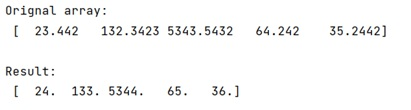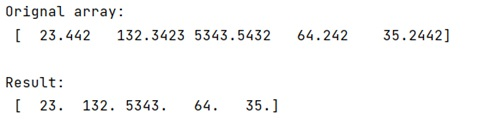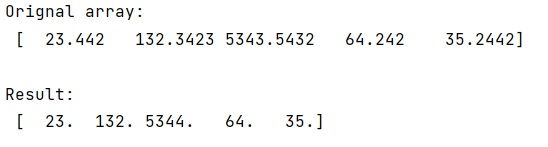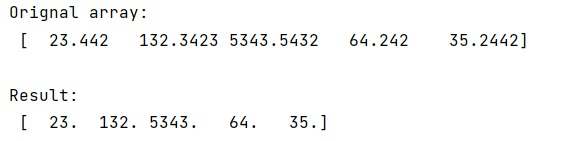# Convert float array to int array in NumPy

In this tutorial, we will learn how to convert float array to int array in NumPy? By Pranit Sharma Last updated : May 23, 2023

Suppose that we are given a two-dimensional NumPy array that contains some float values and we need to convert these float values into int values.

## Converting float array to int array in NumPy

To convert 2D float array to 2D int array in NumPy, we can use the astype() method for changing the data type of the values. However, we have some NumPy functions to control the rounding of the values, for example, rint(), floor(), trunc(), ceil().

We will understand the conversion of float values to int values with the help of each of these methods.

## Convert float array to int array using ceil() method

```import numpy as np

# Creating a numpy array
arr = np.array([23.442,132.3423,5343.5432,64.242,35.2442])

# Display original array
print("Orignal array:\n",arr,"\n")

# Using ceil() method
res = np.ceil(arr)

# Display result
print("Result:\n",res)
```

Output:## Convert float array to int array using trunc() method

```# Import numpy
import numpy as np

# Creating a numpy arrray
arr = np.array([23.442,132.3423,5343.5432,64.242,35.2442])

# Display original array
print("Orignal array:\n",arr,"\n")

# Using trunc method
res = np.trunc(arr)

# Display result
print("Result:\n",res)
```

Output:## Convert float array to int array using rint() method

```# Import numpy
import numpy as np

# Creating a numpy array
arr = np.array([23.442,132.3423,5343.5432,64.242,35.2442])

# Display original array
print("Orignal array:\n",arr,"\n")

# Using rint() method
res = np.rint(arr)

# Display result
print("Result:\n",res)
```

Output:## Convert float array to int array using floor() method

```# Import numpy
import numpy as np

# Creating a numpy array
arr = np.array([23.442,132.3423,5343.5432,64.242,35.2442])

# Display original array
print("Orignal array:\n",arr,"\n")

# Using floor() method
res = np.floor(arr)

# Display result
print("Result:\n",res)
```

Output: